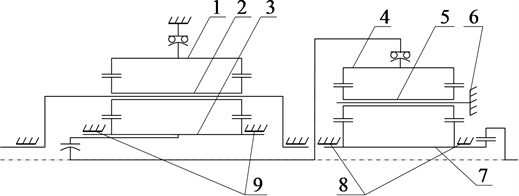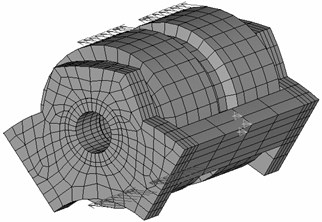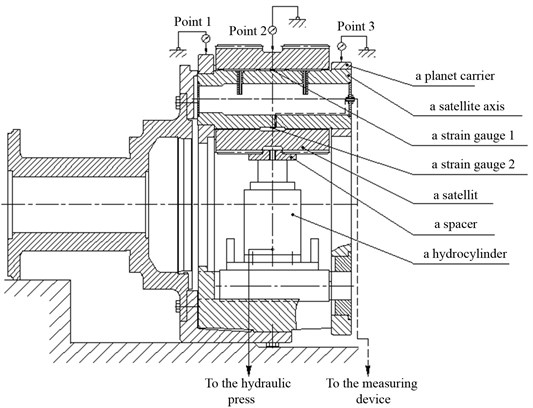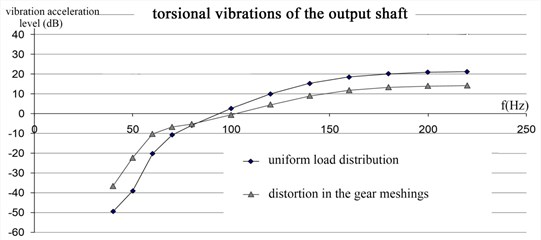Published: 25 June 2019

# The calculation of skews in planetary transmissions and their influence on the system dynamics

Dmitry Nasonov1
Mikhail Leontiev2
1Mechanical Engineering Research Institute of the Russian Academy of Sciences, Moscow, Russian Federation
2, 3Bauman State Technical University (Kaluga Branch), Kaluga, Russian Federation
Corresponding Author:
Dmitry Nasonov
Views 71

#### Abstract

The construction of mathematical models of the planetary gearbox was considered. The presented models allow to analyze the stress-strain state of individual components and, paying attention to the obtained deformations, to calculate the dynamic characteristics of the gearbox taking into account the static load. It was found that the asymmetry of the satellite nodes leads to distortions in the gear meshing. The influence of the available distortions in the meshes on vibroacoustic characteristics was investigated. The design solution to compensate the existing asymmetries and to eliminate the distortions in the meshes was proposed.

## 1. Introduction

As the gearbox is one of the main sources of vibration, the calculation of its dynamic characteristics is of a particular interest. This is confirmed by a large number of publications on the topic [1-3].

In modern marine power plants megasattelite planetary gears are of great use. The fundamental researches in kinematics [4-6] have shown the possibility of achieving sufficiently high values of their efficiency, as well as the advantages in weight and size characteristics in comparison with bulky ones. Many works are devoted to the methods of calculating the natural oscillations of planetary gears.

At the same time, the use of planetary mechanisms in heavily loaded transmissions has set a serious task of equalizing the power transmitted through parallel flows. The problem was solved by using special equalizing mechanisms [6-8] and increasing the support flexibility of the satellites [4, 9].

The use of such solutions complicates both the design itself and the method of its calculation, and this can be applied as to the problems of static loading, as well to the problems of dynamics [2, 10-12]. Besides, it should be mentioned that to solve the problems of dynamics it is necessary, as a rule, to find a solution of a number of static problems to obtain the parameters of a dynamic model.

## 2. A kinematic scheme

The article deals with a two-stage planetary gearbox, the scheme of which is shown in Fig. 1. The high-speed gear stage has three satellites (5) and a fixed planet carrier (6). The low-speed stage has five satellites (2) and a fixed epicycle (1). The solar gears (3, 7) are installed in the floating sliding bearings (8, 9). In order to equalize the load on the satellites, the stiffness of their axes is specially reduced.

Fig. 1The scheme of a two-stage planetary gearbox## 3. The main tasks and methods of their solution

In the study of dynamics, small oscillations of the system are often considered near some equilibrium state which is occupied by the system, as the result of the influence of static components of the force action. Small amplitude of the dynamic perturbations in the gear meshes, relatively to the static load, allows us to consider such oscillations in a linear formulation that makes the task much easier.

The search for the equilibrium state, relatively to which the oscillations are performed, is a sophisticated static problem taking into account the contact interaction of the axes with the planet carrier and with the satellites . Sometimes it is necessary to pay attention to the manufacture and assembly errors, the definition of which is a difficult task too .

Thus, it is necessary to solve two problems. First of all, to calculate the deformed state of the system by applying the static component of the load and taking into account the available nonlinear characteristics. Secondly, to determine the parameters of the linearized model and disturbing forces for the study of dynamic characteristics according to the obtained solution. The main point to be determined is the amplitude-frequency characteristic of vibrations at the control points caused by the process of overexertion of the teeth.

Both problems were solved by means of the ANSYS software complex.

Preliminary studies have allowed to choose the optimal parameters of the finite element grid in terms of the ratio of computing resources and the accuracy of calculations. A finite element model of a satellite node with a minimum number of elements, where the model adequacy is still ensured, is shown at Fig. 2. When solving problems with static load, it was found that the satellite axes are subjected to the effect of ovalization, and part of the load caused by the bending of the axis is perceived by the satellite. This also increases the effect of nonlinearity in calculations. The constructed model adequacy was confirmed by experimental studies on the stand shown in Fig. 3. The radial load was applied to the satellites via the spacer with the help of the hydraulic cylinder. The displacements of the control points 1, 2, 3, as well as the stresses from the load cells 1, 2 were compared with the results of calculations.

Fig. 2A Finite element model of the satellite node (fragment of the carrier and the satellite)Fig. 3The scheme of the experimental standIn contrast to the stand, the developed mathematical model allowed to study deformations not only at radial but also at tangential static load. In the result of the given researches, a negative effect was detected. The developed design of the satellite axis really reduces the unevenness of the load distribution on the satellites, but a skew in the gearing teeth appears. This effect is caused by the fact that the design of the satellite node is not symmetrical with the respect to the central plane which is perpendicular to the satellite axis . This can be seen at Fig. 3. According to preliminary estimates, this effect leads to an increase in local contact stresses in the gear meshes by about 8 %.

As the result, the above effect leads to a redistribution of the dynamic components of the disturbing forces in the meshes, which is important for modeling the force effect in a dynamic model.

## 5. The construction of amplitude-frequency characteristics

To build the amplitude-frequency characteristics at the Ansys Parametric Design Language a special program of designing a parametric model of both the satellite node and the gearbox was written. Some geometric dimensions and the nature of the distribution of disturbing forces were used as the parameters. The calculations were made in a linear formulation as a response to harmonic excitation. The amount of vibration caused by overexertion of teeth in the engagements of the low speed stage of the gearbox in the frequency range 40-230 Hz, was studied. Fig. 4 shows the amplitude-frequency characteristics of the level of torsional vibrations on the output shaft of the gearbox.

Fig. 4Amplitude-frequency characteristics of the gearbox.The Fig. 4 shows that the influence of unevenness of the disturbing forces caused by the skewing of gears is not simple. At frequencies below 80 Hz skewing in gearing leads to an increase of vibration, and at frequencies above 80 Hz it leads, on the contrary, to a decrease. Changing the of vibration level can reach 10-20 dB. The similar characteristics were obtained for transverse and axial oscillations. As well as for torsional vibrations, in the presence of skew the vibration level increases at low frequencies and decreases at high ones. Only the point of intersection of the graphs is shifting to 50 Hz for transverse vibrations and to 100 Hz for the axial oscillations.

However, taking into account the increase in contact stresses, one should not try to reduce the vibration level in the high-frequency part of the range this way. It is more advisable to eliminate the existing distortions. It is shown at Fig. 3 shows that the satellite axis has an internal groove with a changing diameter. To compensate the rigid asymmetry of the satellite node and to eliminate the skew, it is enough to shift the change point of the groove diameter.

## 6. Conclusions

Within the given work, a unique three-dimensional finite element model of a two-stage planetary gearbox was designed, which allowed to study the influence of various equalizing devices on the deformed state of all components of the gearbox.

The redistribution of disturbing forces caused by a skew in the gear meshing has been investigated.

New knowledge about the influence of distortions in the gear meshings of a typical planetary gearbox on its dynamic characteristics has been obtained.

The recommendations to improve the design of the satellite node of the planetary gearbox have been given.